# OASP笔记 - 3

## 光谱仪

Posted by mingjie on December 18, 2017

### 概述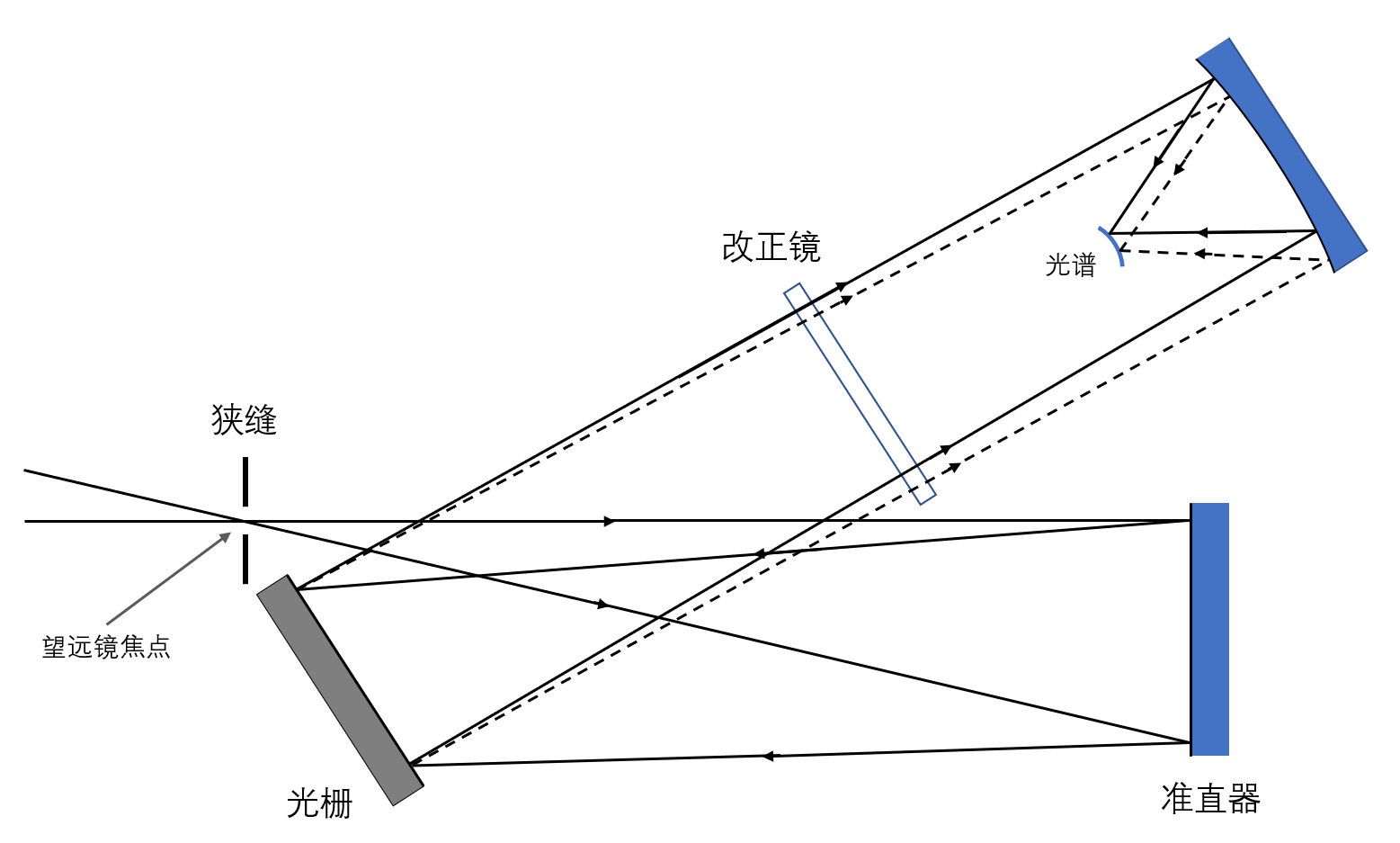光谱仪示意图

### 衍射光栅及其原理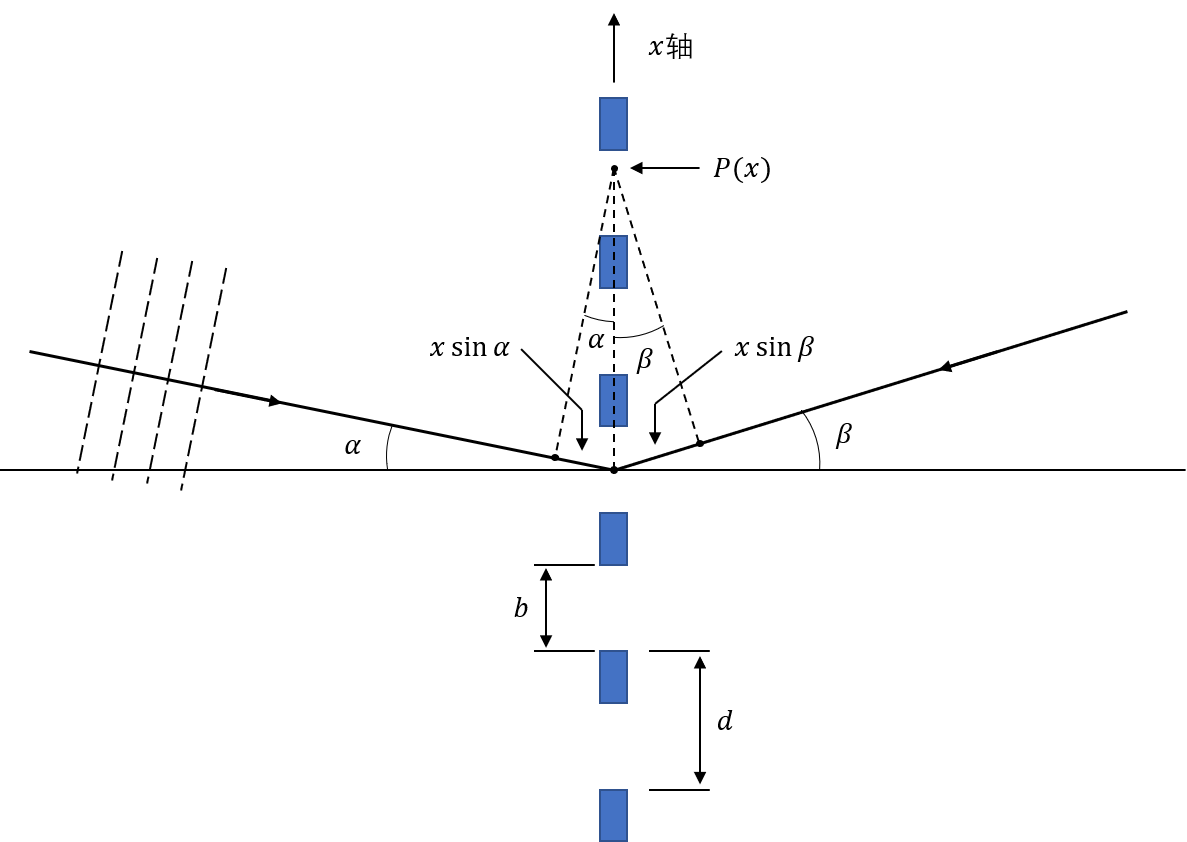衍射光栅示意图

$P(0)$所指示的点为相位0点，则在光栅上任一点和它的相位差为$x \sin{\alpha}$$\alpha$为入射平面光法向与光栅法向的夹角，是一个定值。所以我们可以将在光栅上的入射光表达为：

$G(x)$为光栅，

$\beta$角上看到的光强为$F(x, t)$$G(x)$的乘积加上离开光栅后的相位差在$x$上的积分：

$\theta = \frac{\theta'}{\lambda} = \frac{\sin{\alpha} + \sin{\beta}}{\lambda}$，有

#### $G(x)$的傅里叶变换

$G(x)$是由宽为$b$的狭缝等距$d$摆放直至充满宽度$W$而来的：

$B_1(x)$为单个狭缝，它与$III(x)$的卷积为无限宽的光栅，$B_2(x)$为光栅的宽度。它的傅里叶变换为：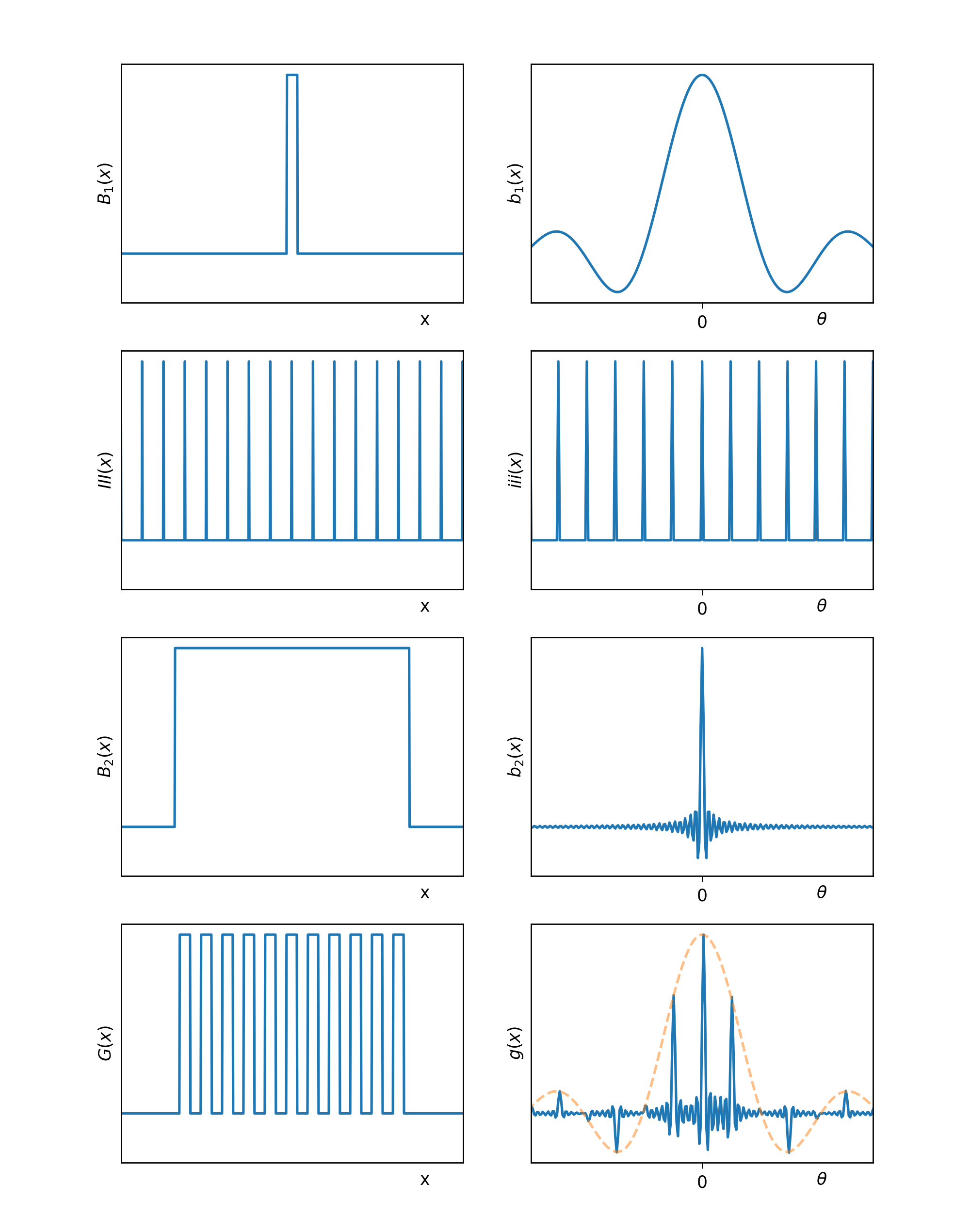光栅以及它的傅里叶变换

$\Delta \theta' = \frac{\lambda}{W}$代入$(3.6)$，得：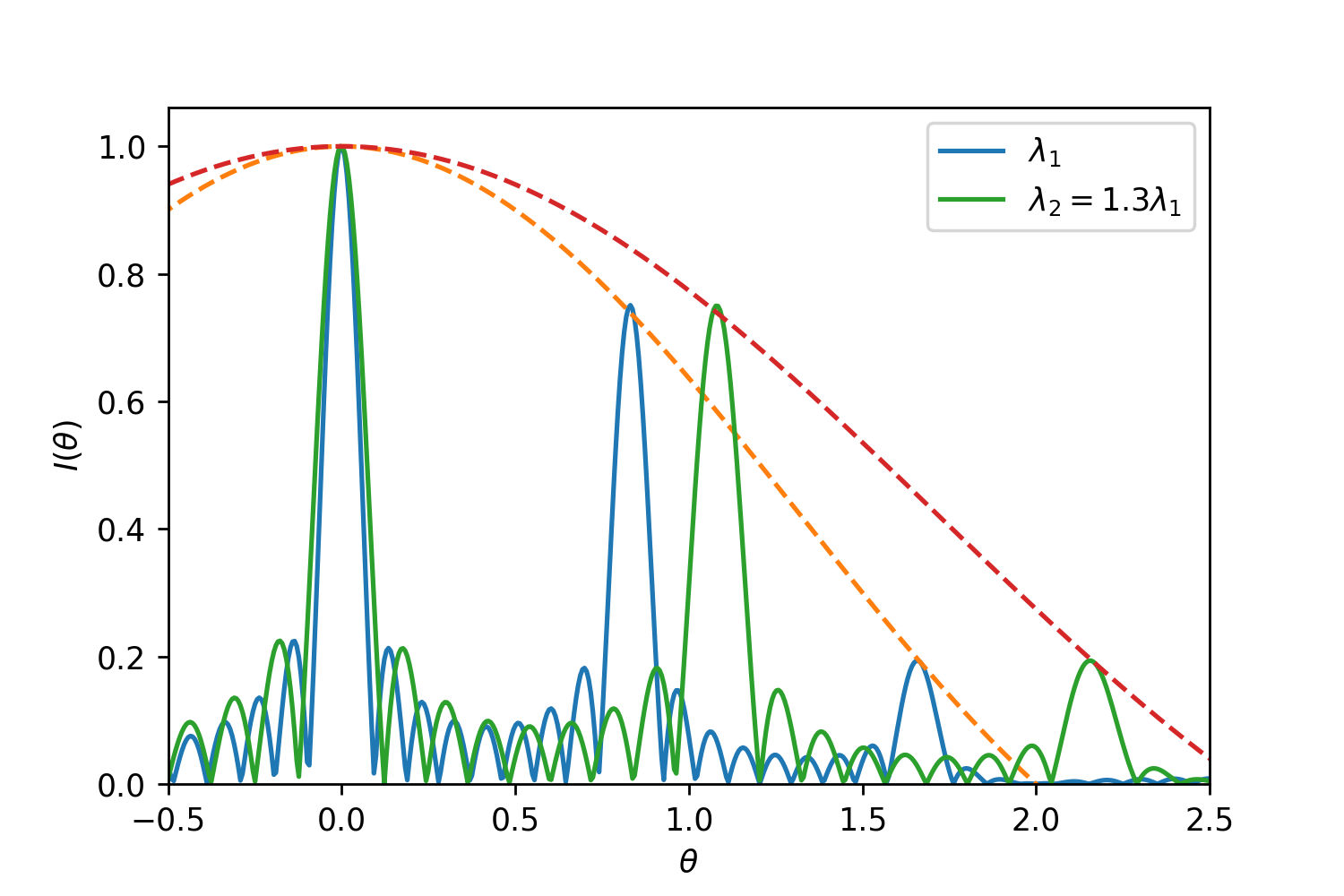复色光入射时的情况

### 闪耀光栅闪耀光栅（右）示意图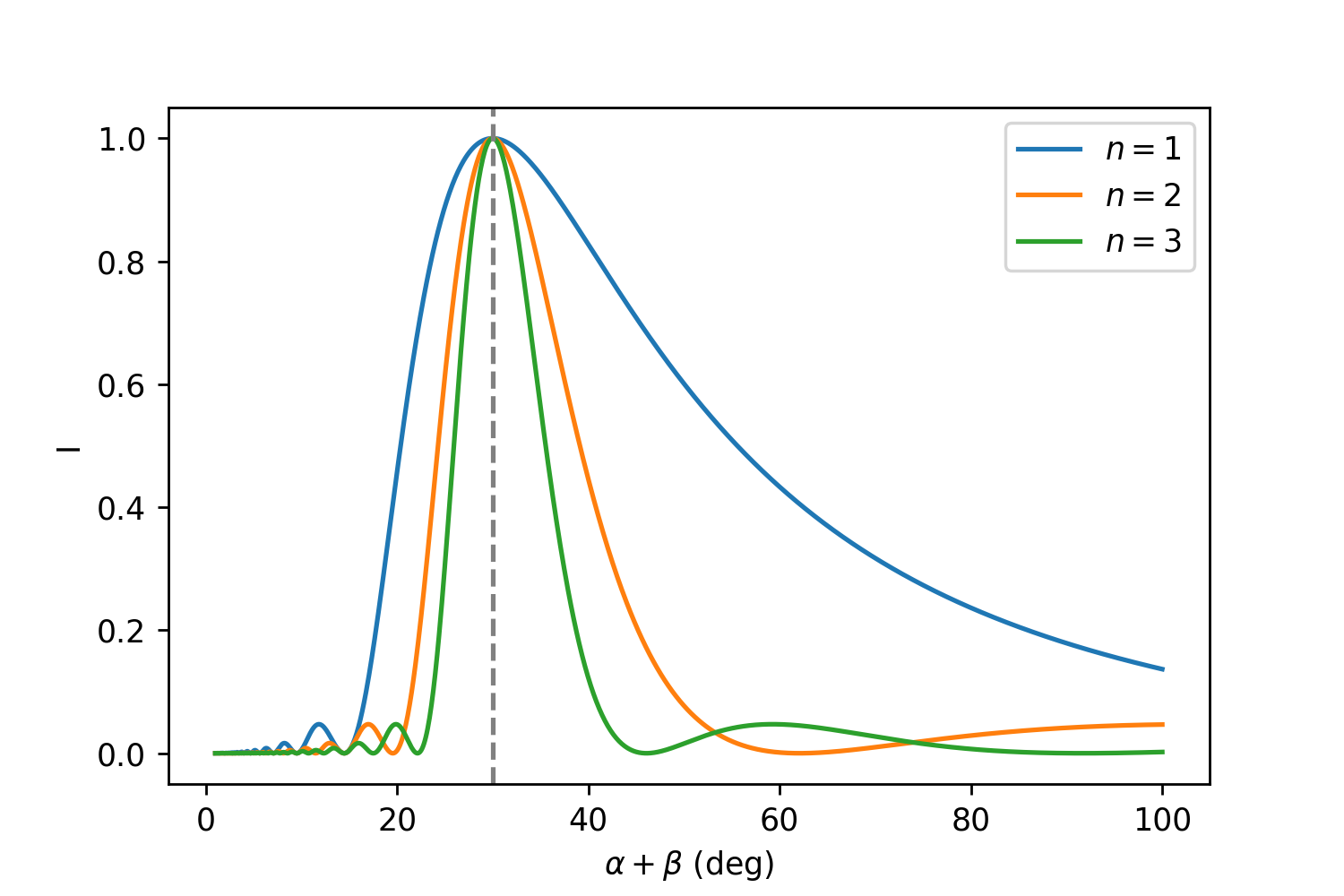闪耀光栅的光强包络线，$\phi=15^\circ$

### 色散和分辨率

#### 角、线色散#### 分辨率

$(3.5)$式求导，得出：

$w$为狭缝在CCD上的像宽，则

${w/W'}$正比于$f_\mathrm{cam}/f_\mathrm{coll}$。一般来说我们需要CCD的像素与狭缝在CCD上的像宽相匹配，最好是1个单位像宽对应CCD上的两个像素（奈奎斯特频率）。稍微的过采样可能会有好处，但是面临着更长时间曝光和深度减小的问题；而欠采样会使得信息丢失。

### 阶梯光谱仪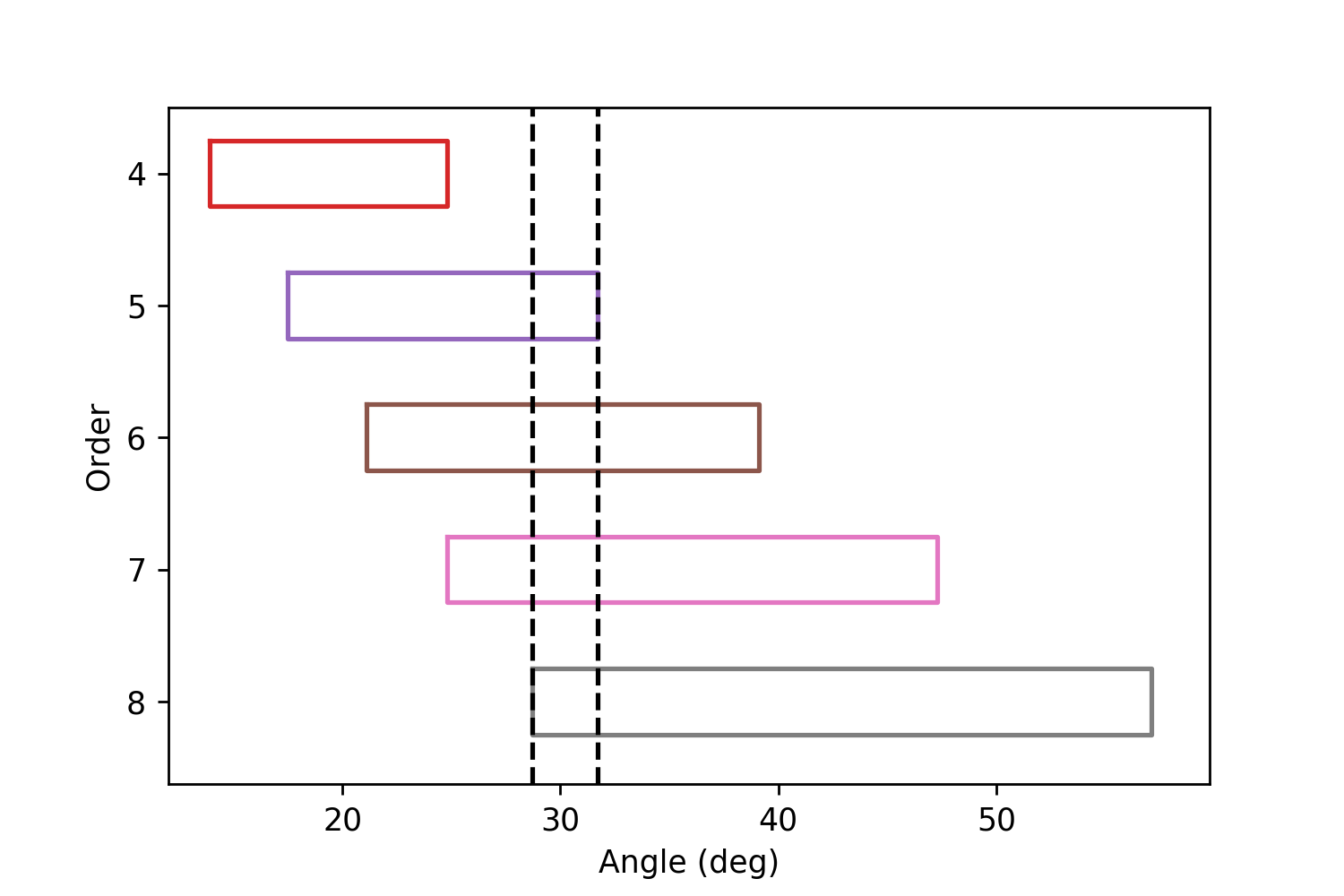高级数光谱重叠情况；选择的当的话黑色虚线中间可以包含了整个波长范围的光阶梯光谱仪示意图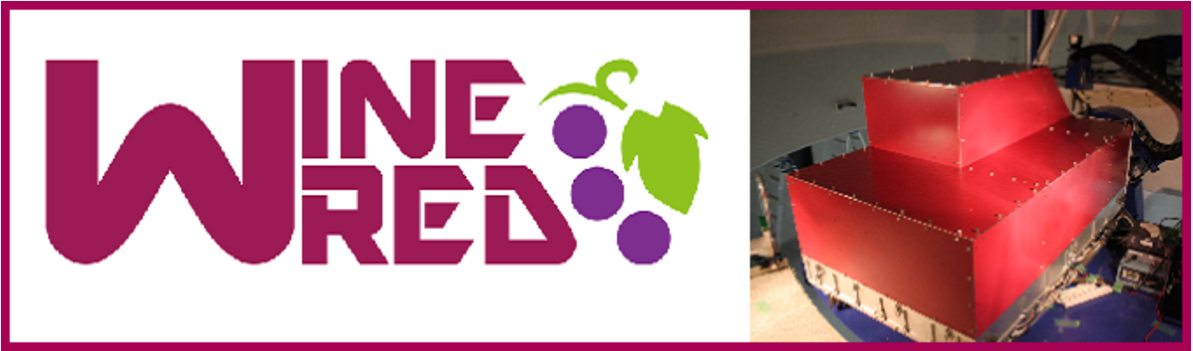WINERED与其他光谱仪参数对比

### 略过的内容